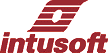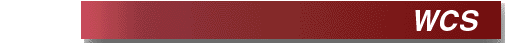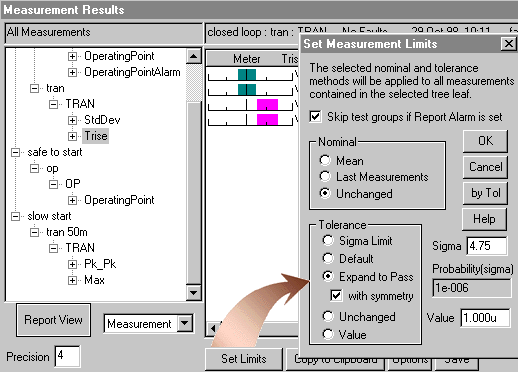WCS, Worst Case by Sensitivity In this analysis (actual WCS.SCP file), we first run a reference simulation and make a plot to save the results. Then a new simulation is run for each toleranced parameter, perturbing the parameter by a fraction of its tolerance. Then the differences between these measurements and the reference measurements are saved. The absolute value of the difference measurements are summed and saved in the IsSpice4 output file and in a format that can be read back into the Results dialog in SpiceNet. This is not as rigorous as an EVA analysis or a worst case by optimization; however, it is the most computationally efficient method. You can set the min-max measurement test limits by expanding the measurements to pass with symmetry in the Results dialog; as shown below.The WCS analysis is based on the assumption that each measurement is a linear function of all of the parameters. For most moderately complex circuits this assumption is invalid. Generally you will get tolerances larger than the 3 sigma limits of a statistical analysis, however, you should run a Monte Carlo analysis for at least 6 cases and set tolerances based on the Monte Carlo analysis, usually to 5 sigma, before expanding the WCS data in order to be sure that non-linear relationships are taken into account.